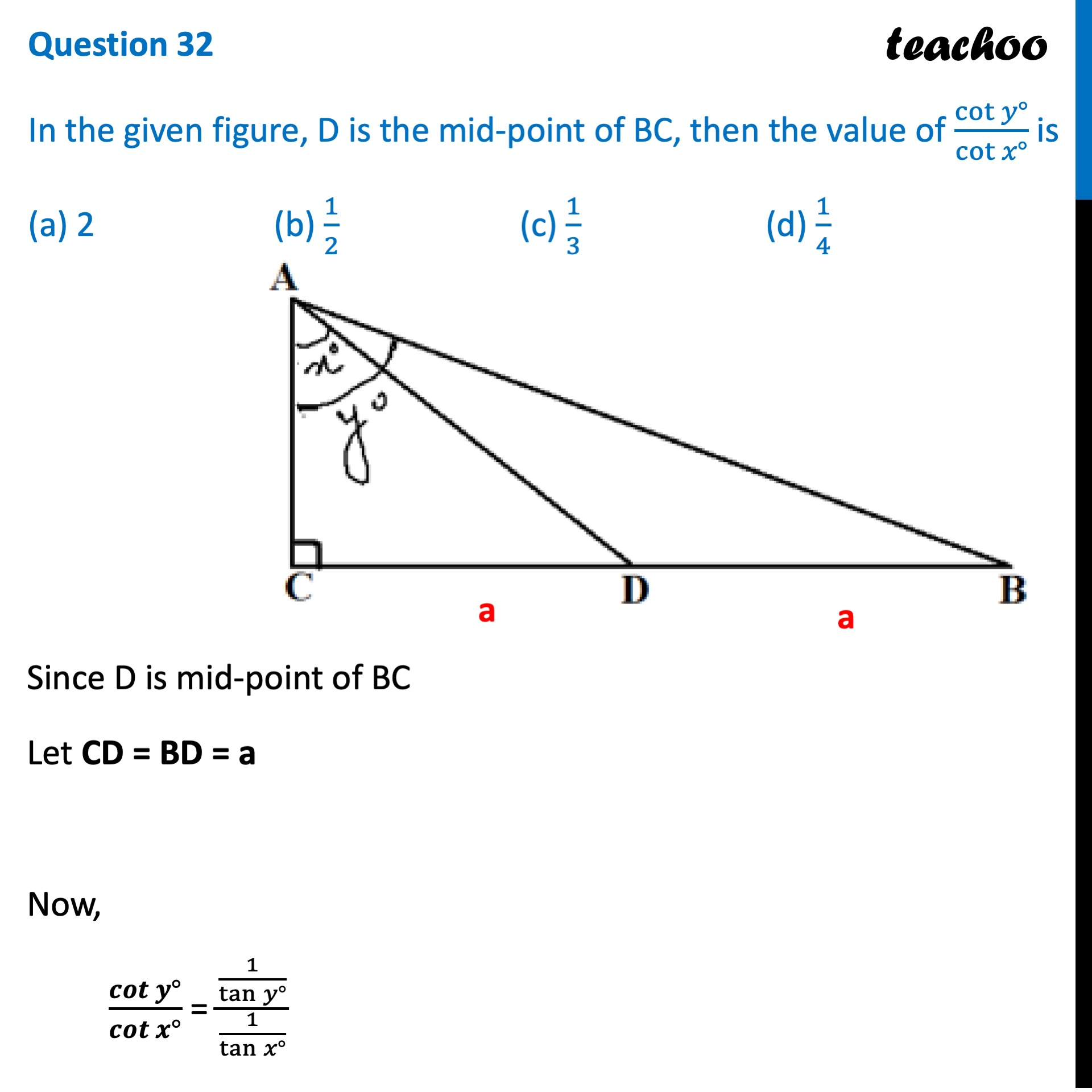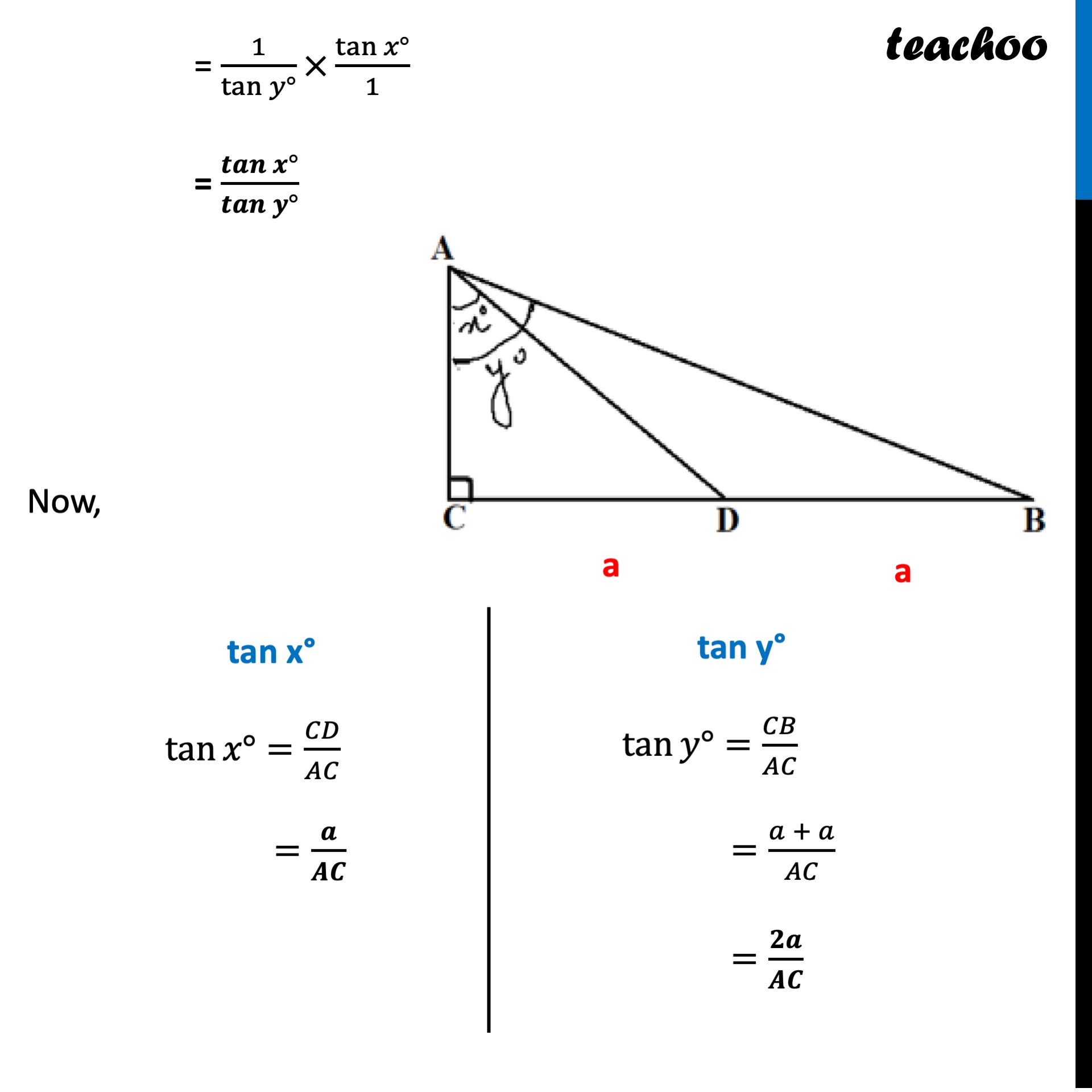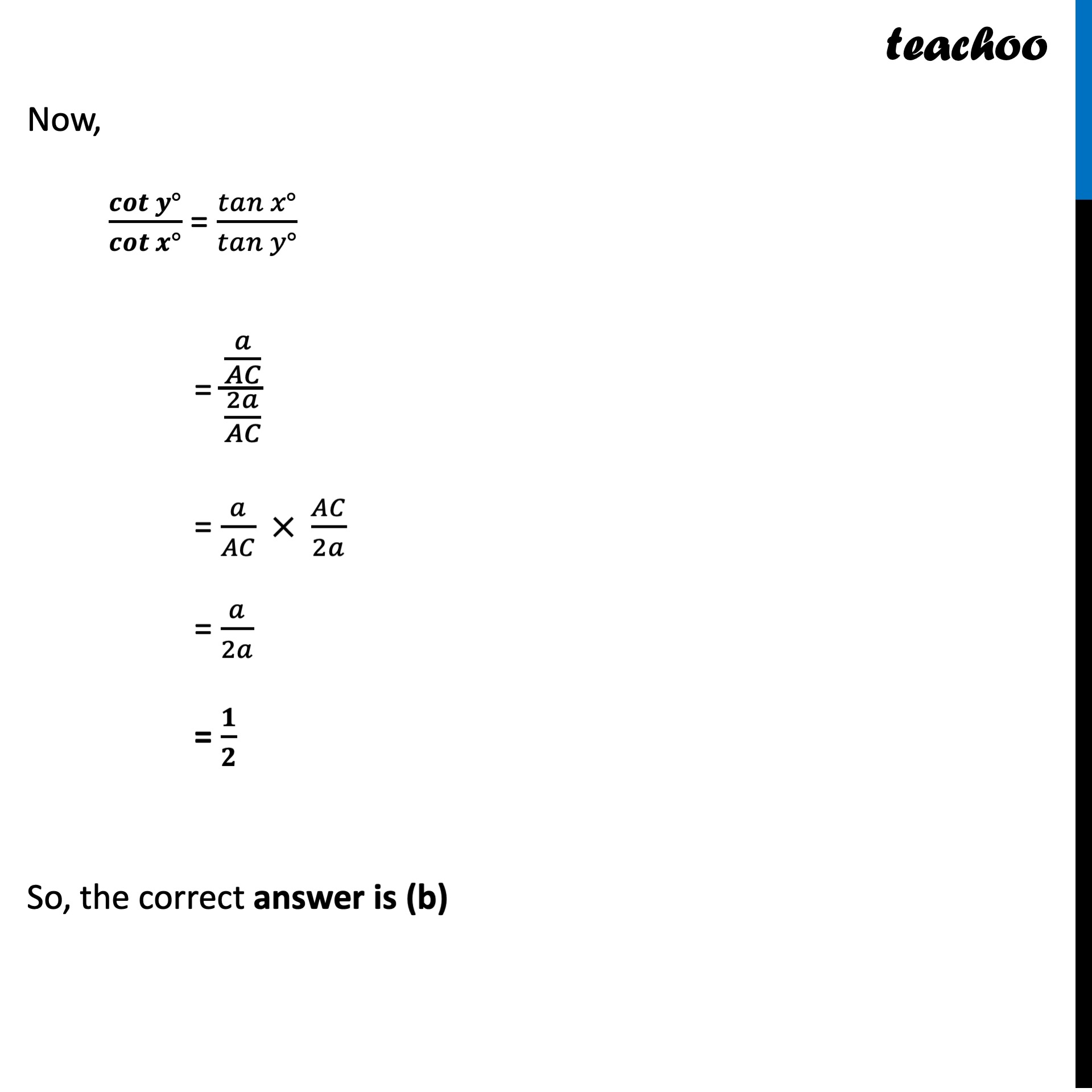##Advertisement (adsbygoogle = window.adsbygoogle || []).push({});Advertisement (adsbygoogle = window.adsbygoogle || []).push({});1. Class 10
2. Solutions of Sample Papers for Class 10 Boards
3. CBSE Class 10 Sample Paper for 2022 Boards - Maths Standard [MCQ]

Transcript

Question 32 In the given figure, D is the mid-point of BC, then the value of cot 𝑦 ° cot 𝑥 ° is (a) 2 (b) 1/2 (c) 1/3 (d) 1/4 Since D is mid-point of BC Let CD = BD = a Now, 𝒄𝒐𝒕⁡〖𝒚°〗/𝒄𝒐𝒕⁡〖𝒙°〗 = (1/tan⁡〖𝑦°〗 )/(1/tan⁡〖𝑥°〗 ) = 1/tan⁡〖𝑦°〗 ×tan⁡〖𝑥°〗/1 = 𝒕𝒂𝒏⁡〖𝒙°〗/𝒕𝒂𝒏⁡〖𝒚°〗 Now, tan x° tan⁡〖𝑥°〗=𝐶𝐷/𝐴𝐶 =𝒂/𝑨𝑪 tan y° tan⁡〖𝑦°〗=𝐶𝐵/𝐴𝐶 =(𝑎 + 𝑎)/𝐴𝐶 =𝟐𝒂/𝑨𝑪 Now, 𝒄𝒐𝒕⁡〖𝒚°〗/𝒄𝒐𝒕⁡〖𝒙°〗 = 𝑡𝑎𝑛⁡〖𝑥°〗/𝑡𝑎𝑛⁡〖𝑦°〗 = (𝑎/𝐴𝐶)/(2𝑎/𝐴𝐶) = 𝑎/𝐴𝐶 × 𝐴𝐶/2𝑎 = 𝑎/2𝑎 = 𝟏/𝟐 So, the correct answer is (b)